﻿ExprBinary Fields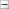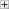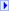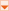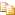The ExprBinary type exposes the following members.

#Fields

NameDescriptionDIVIDE
Division operator.LOG
Logarithm operator.MINUS
Subtraction operator.MOD
Modulo (remainder) operator.MULTIPLY
Multiplication operator.PLUS
Addition operator.POWER
Exponentiation operator.ROUND
Round operator.TRUNC
Truncation operator.